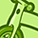# New to Qlik Sense

If you’re new to Qlik Sense, start with this Discussion Board and get up-to-speed quickly.

Announcements
cancel
Showing results for
Did you mean:Contributor

## Cumulative Value over periods in Combo Chart

I have a combo chart with bar charts showing delta's actual Vs Budget per month.
I want to add a line showing the cumulative differences for the year.
As a newbie I tried with Aggr(RangeSum(Above several times and based upon different suggestions I found in the forum. However I can't make it work.
I'm using below expresion for the delta calculation:

(-Sum({<CY={'1'}, [AccCode] = {2} ,[P&L/Balance]={'P&L'}>}-[#Actual]) +
Sum({<CY={'1'}, [AccCode] -= {2} ,[P&L/Balance]={'P&L'}>}[#Actual]))/1000
-
(-Sum({<CY={'1'}, [AccCode] = {2} ,[P&L/Balance]={'P&L'}>}-[#Budget]) +
Sum({<CY={'1'}, [AccCode] -= {2} ,[P&L/Balance]={'P&L'}>}[#Budget]))/1000

Anyone able to help me out on how to create a cumulative line for these delta's?

I tried starting above with :

Aggr(RangeSum(Above

& ending with:

, 0, RowNo())), (\$(vJYearMonthNr), (NUMERIC)))

Labels (2)

• ### General Question

1 Solution

Accepted SolutionsMVP

Something like this?This is what I used

``````=Aggr(
RangeSum(Above(TOTAL
Sum({<cytd = {'1'}, acccode = {'2'}, [b/p&l] = {'P&L'}>} actual) - Sum({<cytd = {'1'}, [acccode] = {'2'}, [b/p&l] = {'P&L'}>} [budget])
, 0, RowNo()))
, \$(='['& vYearMonthNr & ']'))``````
8 RepliesMVP

What is your variable vJYearMonthNr here? Is it giving a field or expression?Contributor
Author

This variable is giving a field.MVP

May be try this

``````\$(='[' & \$(vJYearMonthNr) & ']')

or

\$(='[' & vJYearMonthNr & ']')``````Contributor
Author

Hi Sunny,

Thanks for your suggestion.

However. Unfortunately both suggestions don't seem to work and results in not showing any data at all. Is there any other way to get the cumu line in this graph?MVP

Would you be able to share a sample to look at the issue?Contributor
Author

Unfortunatly I am not able to share the original file.

I recreated some data in an excel file and created the visualisation. In the formula editor I incorporated several tries and suggestions but also don't seem to work in this test file.MVP

Something like this?This is what I used

``````=Aggr(
RangeSum(Above(TOTAL
Sum({<cytd = {'1'}, acccode = {'2'}, [b/p&l] = {'P&L'}>} actual) - Sum({<cytd = {'1'}, [acccode] = {'2'}, [b/p&l] = {'P&L'}>} [budget])
, 0, RowNo()))
, \$(='['& vYearMonthNr & ']'))``````Contributor
Author

Yes, Thanks so much! This works